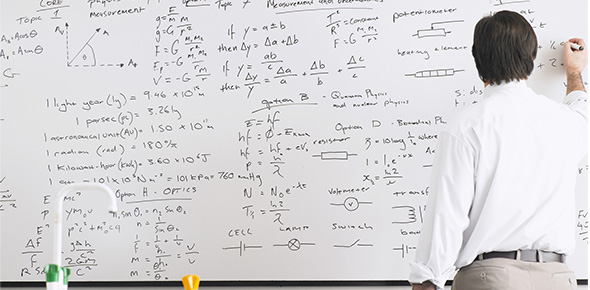# Solving Systems Of Linear Equations - Mr. Bell

12 Questions | Total Attempts: 20SettingsThis quiz will test your knowledge of solving systems of linear equations.

• 1.
c = 13x + 17c = -3x + 1Solve for x
• 2.
C = 13x + 17c = -3x + 1Use the previous question/answer to solve for c
• 3.
j = 45 - 5aj = 35 - 7aSolve for a
• 4.
J = 45 - 5aj = 35 - 7aUse the previous question/answer to solve for j
• 5.
e = 15 + 9ke = 20 + 4kSolve for k
• 6.
e = 15 + 9ke = 20 + 4kUse the previous question/answer to solve for e
• 7.
y = 700 - 0.5xy = 900 - 1xSolve for x
• 8.
y = 700 - 0.5xy = 900 - 1xUse the previous question/answer to solve for y
• 9.
y = 34x - 29y = 13x + 13Solve for x
• 10.
y = 34x - 29y = 13x + 13Use the previous question/answer to solve for y
• 11.
h = 2t - 6h = 15 + 0.25tSolve for t
• 12.
h = 2t - 6h = 15 + 0.25tUse the previous question/answer to solve for h
Related TopicsBack to top